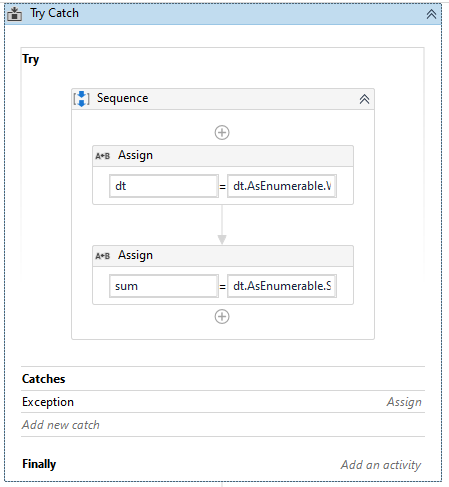# Adding data from a filtered column

I have a table with the columns “CEDULA”, “IBCS” and “SALUD”, I must filter the cases in which “SALUD” is equal to “XXXX” and in “IBCS”, how can I do that?
I have this so far

the value of that sum must be stored in a variable
Regards,
@ppr
@supermanPunch
@Seetharaman_K
@ClaytonM

1 Like

Hello Beatriz

The easiest way is to use the activity ‘Filter Data table’.The above screenshot shows what you’re looking forTry to tick the option ‘Keep’ and can you share the data in dt2?

If you can’t get the Filter activity to work, here is an alternative way using .net

``````Assign:
filteredRows = dt2.Select.Where(Function(r) r("SALUD").ToString.Trim.ToUpper="EPS010" And If(IsNumeric(r("IBCS").ToString.Trim), CLng(r("IBCS").ToString.Trim), False) ).ToArray

IF activity, condition filteredRows.Count > 0
Assign: Sura = filteredRows.Sum(Function(r) CLng(r("IBCS").ToString.Trim) )
``````

using the filtered rows variable, you can update the rows while keeping the entire data set. However, you can store those filtered rows to a new table with filteredRows.CopyToDataTable inside the IF activity.

I also suggest using CLng() rather than CInt() since the numbers are larger.

If the filtered rows is giving you a .Count of 0, still, then there could be something different with our excel file that we aren’t considering.

Is the column IBCS in Int32 format?

1 Like

Hi!
`try {`
`dt = dt.AsEnumerable.Where(Function (row) row("SALUD").ToString.Equals("EPS010") and CDbl(row("IBCS").ToString) <8778020).CopyToDataTable`

`sum = dt.AsEnumerable.Sum(Function (row) CDbl(row("IBCS").ToString))`
`} catch (Exception e) {`
//your filtered table has no rows
`sum = 0`
`}`1 Like

But and the condition of only taking the values of the “IBCS” in which they are minorea to 8778020

Yes, is int32

Yes, I made a mistake. It should be < in the condition:

``````filteredRows = dt2.Select.Where(Function(r) r("SALUD").ToString.Trim.ToUpper="EPS010" And If(IsNumeric(r("IBCS").ToString.Trim), CLng(r("IBCS").ToString.Trim) < 8778020, False) ).ToArray
``````Regards

It is not very clear to me how I should generate the Try Catch

But I am confused in the catches

I’m not sure if I put the structure right

That is assigning it as an Array(Of DataRows), so you will need to assign that to that type of variable, not a data table.

The reason you must do this, is because if it filters to ‘no’ datarows, it will throw an exception if you try to use .CopyToDatable (as seen by the previous version of this solution.

If you take a look at my earlier post, it shows how you will need to use an IF activity to check that it found any DataRows. Then, you can do any actions on that array (preferred) or use .CopytoDatable if you wish.

I don’t recommend using a Try/Catch in this method. Normally, it is better to use IF activity to check that the data is valid before calculating.

For example, checking that the filter found any data rows before converting to a datatable or calculating its sum, as shown in my earlier post with an example of assigning the filtered set to an Array(Of DataRows), then using .Count>0 as a condition.

Hope this helps you further.

Regards.

Catch block will be executed when your filtered table has no rows.
You can put there any logic you want.
E.g. Assign sum = 0

I’ll post a xaml of the filter working in a bit for you.

Totales(filterpart).xaml (10.0 KB) Totales(filterpart2).xaml (10.0 KB)

This is just the filter part. I posted 2 versions, one using the Filter Data Table activity and one using .net. So, you have both

Apologies, most of it is in English.

Turns out, the Filter Data Table activity has a problem with matching strings together. Even when I used ‘Starts With’ combined with ‘Ends With’, it still did not work. However, ‘Starts With’ by itself works fine.

Alternatively, you have the .net version as well in case you like it better. For some reason, ‘IsNumeric()’ was causing a problem, so I changed it to ‘Integer.TryParse()’, as you can see in the xaml.

I placed Write Lines to output the values after the calculation for testing. When I ran it using your criteria, I was seeing 56 rows with sum of 176461845.

I stored the file path and criteria for filtering into variables to make it easier for you.

Let us know if you get further errors around this.

Regards.

1 Like

There is an assign activity in the xaml I posted that adds up all the values in the IBCS column. Check that and let me know if that doesn’t do what you want.

EDIT: also a Write Line that outputs that value

It is perfect.
I am actually very grateful

1 Like

Thanks to your help I managed to filter each one of the data I needed, and I managed to do the rest of the percentages and mathematical processes I needed, but this information that I have already filtered, I can modify these formulas so that I can eliminate or not take into account some users’ rows that correspond to a “CEDULA”?

@ClaytonM

Hi,
Can you clarify your question? Are you wanting to remove duplicated rows?

No, I want to delete some users “CEDULA=‘90850158’” can be the example with this user, not because it is repeated, I just need to delete some users